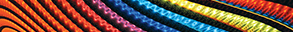Journal metrics
Acceptance rate14%
Submission to final decision40 days
Acceptance to publication54 days
CiteScore1.300
Impact Factor-

### Perona-Malik Model with Diffusion Coefficient Depending on Fractional Gradient via Caputo-Fabrizio Derivative

####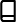Journal profile

Abstract and Applied Analysis publishes research with an emphasis on important developments in classical analysis, linear and nonlinear functional analysis, ordinary and partial differential equations, optimisation theory, and control theory.

####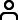Editor spotlight

Chief Editor, Dr Wong, is an associate professor at Nanyang Technological University, Singapore. Her research interests include differential equations, difference equations, integral equations, and numerical mathematics.

####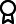Special Issues

Do you think there is an emerging area of research that really needs to be highlighted? Or an existing research area that has been overlooked or would benefit from deeper investigation? Raise the profile of a research area by leading a Special Issue.

## Latest Articles

More articles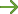Research Article

### Geographically Weighted Multivariate Logistic Regression Model and Its Application

This study investigates the geographically weighted multivariate logistic regression (GWMLR) model, parameter estimation, and hypothesis testing procedures. The GWMLR model is an extension to the multivariate logistic regression (MLR) model, which has dependent variables that follow a multinomial distribution along with parameters associated with the spatial weighting at each location in the study area. The parameter estimation was done using the maximum likelihood estimation and Newton-Raphson methods, and the maximum likelihood ratio test was used for hypothesis testing of the parameters. The performance of the GWMLR model was evaluated using a real dataset and it was found to perform better than the MLR model.

Research Article

### Algorithm for Solutions of Nonlinear Equations of Strongly Monotone Type and Applications to Convex Minimization and Variational Inequality Problems

Real-life problems are governed by equations which are nonlinear in nature. Nonlinear equations occur in modeling problems, such as minimizing costs in industries and minimizing risks in businesses. A technique which does not involve the assumption of existence of a real constant whose calculation is unclear is used to obtain a strong convergence result for nonlinear equations of -strongly monotone type, where . An example is presented for the nonlinear equations of -strongly monotone type. As a consequence of the main result, the solutions of convex minimization and variational inequality problems are obtained. This solution has applications in other fields such as engineering, physics, biology, chemistry, economics, and game theory.

Research Article

### Euler-Type Integral Operator Involving -Function

The object of the present paper is to establish some interested theorems on Euler-type integrals involving -function, which is defined by Saxena and Daiya. Further, we reduce some special cases involving various known functions like the -Mittag-Leffler function, -function, and -series.

Research Article

### On Fuzzy Soft Linear Operators in Fuzzy Soft Hilbert Spaces

Due to the difficulty of representing problem parameters fuzziness using the soft set theory, the fuzzy soft set is regarded to be more general and flexible than using the soft set. In this paper, we define the fuzzy soft linear operator in the fuzzy soft Hilbert space based on the definition of the fuzzy soft inner product space in terms of the fuzzy soft vector modified in our work. Moreover, it is shown that , and are suitable examples of fuzzy soft Hilbert spaces and also some related examples, properties and results of fuzzy soft linear operators are introduced with proofs. In addition, we present the definition of the fuzzy soft orthogonal family and the fuzzy soft orthonormal family and introduce examples satisfying them. Furthermore, the fuzzy soft resolvent set, the fuzzy soft spectral radius, the fuzzy soft spectrum with its different types of fuzzy soft linear operators and the relations between those types are introduced. Moreover, the fuzzy soft right shift operator and the fuzzy soft left shift operator are defined with an example of each type on . In addition, it is proved, on , that the fuzzy soft point spectrum of fuzzy soft right shift operator has no fuzzy soft eigenvalues, the fuzzy soft residual spectrum of fuzzy soft right shift operator is equal to the fuzzy soft comparison spectrum of it and the fuzzy soft point spectrum of fuzzy soft left shift operator is the fuzzy soft open disk . Finally, it is shown that the fuzzy soft Hilbert space is fuzzy soft self-dual in this generalized setting.

Research Article

### Two-Weight, Weak-Type Norm Inequalities for a Class of Sublinear Operators on Weighted Morrey and Amalgam Spaces

Let be a class of sublinear operators satisfying certain size conditions introduced by Soria and Weiss, and let be the commutators generated by functions and . This paper is concerned with two-weight, weak-type norm estimates for these sublinear operators and their commutators on the weighted Morrey and amalgam spaces. Some boundedness criteria for such operators are given, under the assumptions that weak-type norm inequalities on weighted Lebesgue spaces are satisfied. As applications of our main results, we can obtain the weak-type norm inequalities for several integral operators as well as the corresponding commutators in the framework of weighted Morrey and amalgam spaces.

Research Article

### Fixed Point Theorems for a New Class of Mappings in Modular Spaces Endowed with a Graph

In this paper, we discuss a class of mappings more general than -nonexpansive mapping defined on a modular space endowed with a graph. In our investigation, we prove the existence of fixed point results of these mappings. Then, we also introduce an iterative scheme for which proves the convergence to a fixed point of such mapping in a modular space with a graph.Journal metrics
Acceptance rate14%
Submission to final decision40 days
Acceptance to publication54 days
CiteScore1.300
Impact Factor-Author guidelinesEditorial boardDatabases and indexing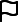Sign up for content alertsSign upAuthor guidelinesEditorial boardDatabases and indexingSign up for content alertsSign up

We are committed to sharing findings related to COVID-19 as quickly and safely as possible. Any author submitting a COVID-19 paper should notify us at help@hindawi.com to ensure their research is fast-tracked and made available on a preprint server as soon as possible. We will be providing unlimited waivers of publication charges for accepted articles related to COVID-19. Sign up here as a reviewer to help fast-track new submissions.